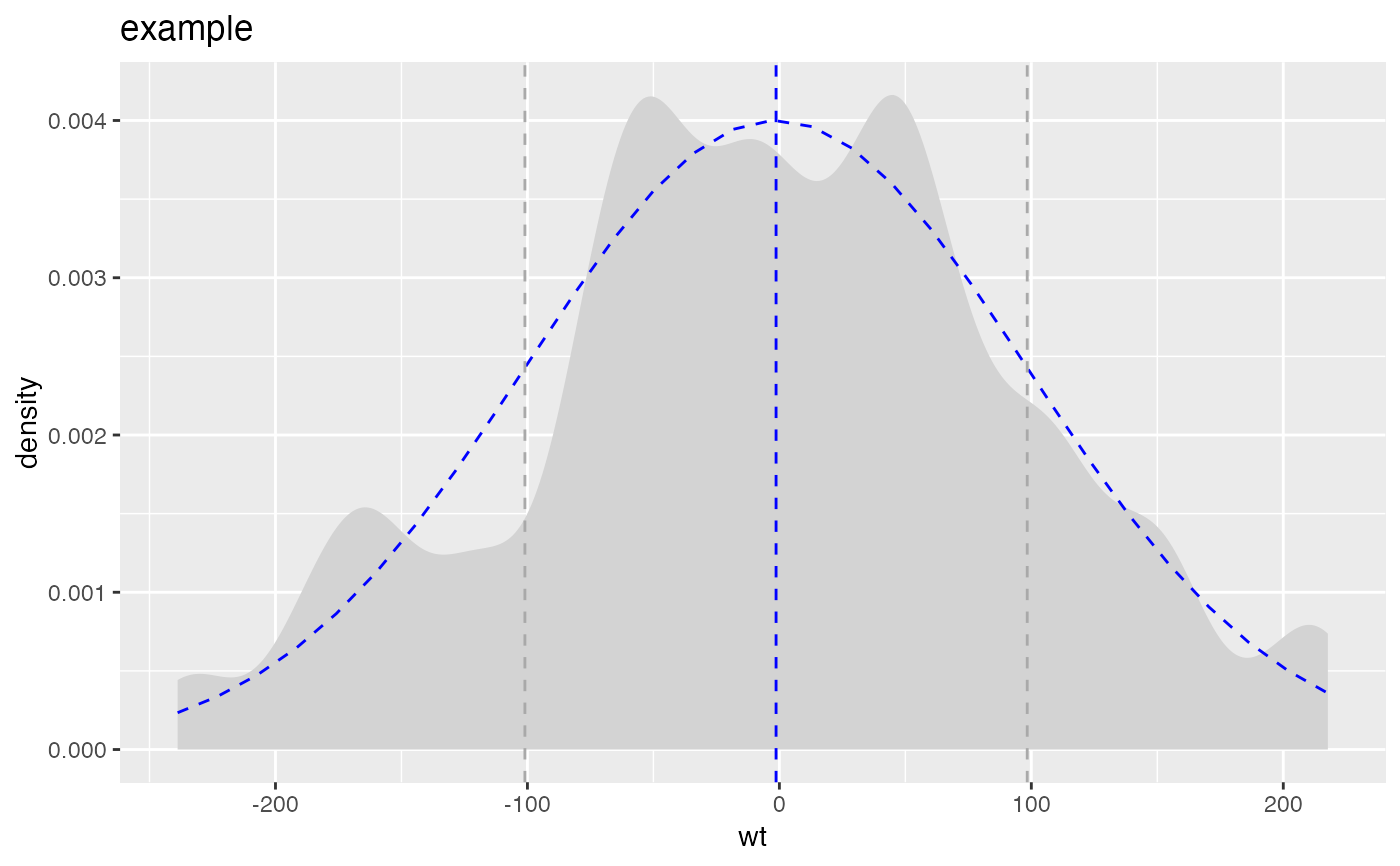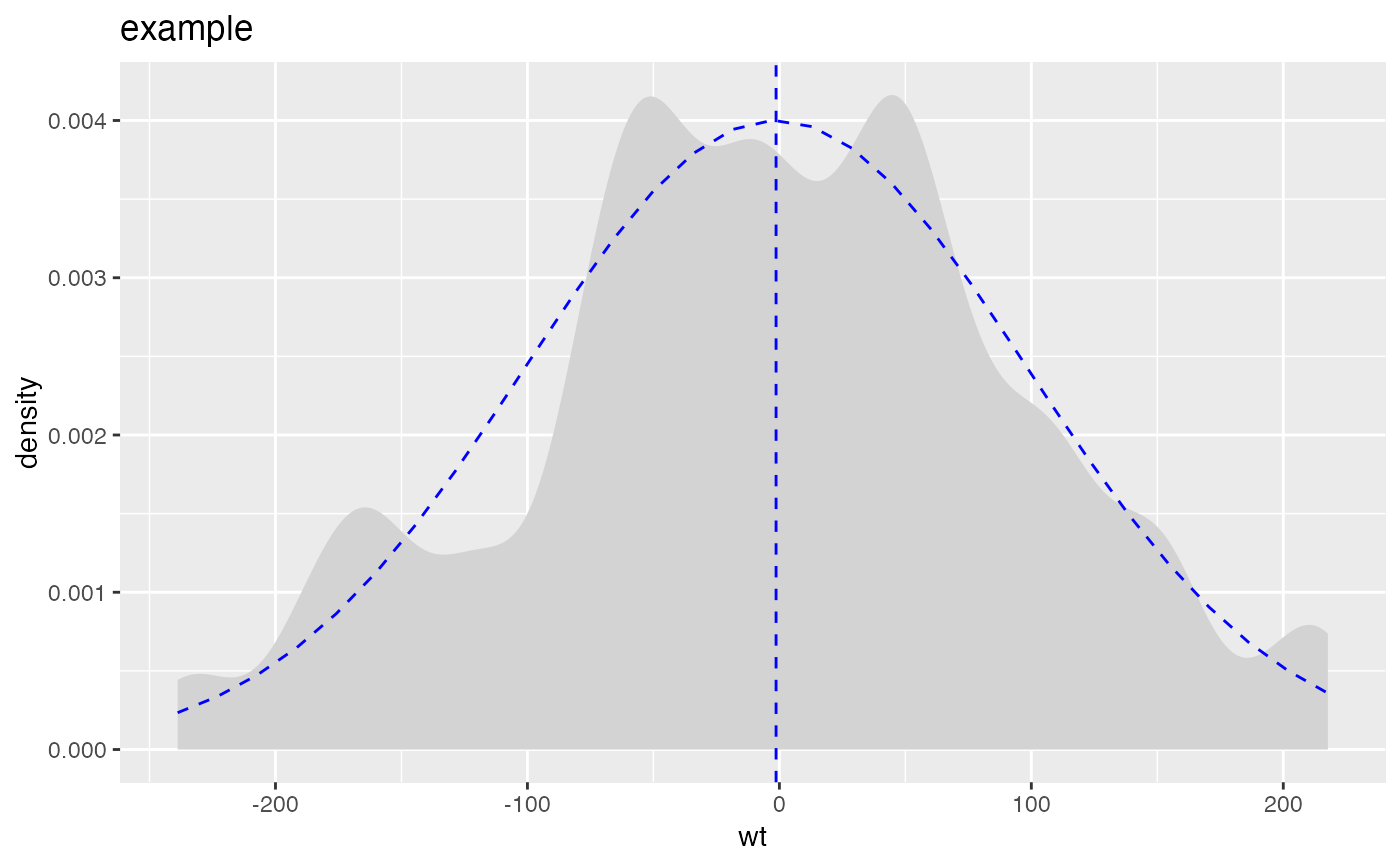Compares empirical data to a normal distribution with the same mean and standard deviation.

PlotDistDensityNormal(
frm,
xvar,
title,
...,
curve_color = "lightgray",
normal_color = "blue",
mean_color = "blue",
sd_color = "darkgray"
)

## Arguments

frm data frame to get values from name of the independent (input or model) column in frame title to place on plot no unnamed argument, added to force named binding of later arguments. passed to geom_density; controls smoothness of density plot color for empirical density curve color for theoretical matching normal color of mean line color for 1-standard deviation lines (can be NULL)

## Details

Plots the empirical density, the theoretical matching normal, the mean value, and plus/minus one standard deviation from the mean.

geom_density

PlotDistDensityNormal(d,'wt','example')PlotDistDensityNormal(d, 'wt', 'example', sd_color=NULL)